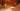# 时间切片（Time Slicing）

## 如何使用时间切片

``````btn.onclick = function () {
someThing(); // 执行了50毫秒
setTimeout(function () {
otherThing(); // 执行了50毫秒
});
};``````

``````btn.onclick = ts([someThing, otherThing], function () {
console.log('done~');
});``````

ES6 带来了迭代器的概念，并提供了生成器 Generator 函数用来生成迭代器对象，虽然 Generator 函数最正统的用法是生成迭代器对象，但这不妨我们利用它的特性做一些其他的事情。

Generator 函数提供了 `yield` 关键字，这个关键字可以让函数暂停执行。然后通过迭代器对象的 `next` 方法让函数继续执行。

``````btn.onclick = ts(function* () {
someThing(); // 执行了50毫秒
yield;
otherThing(); // 执行了50毫秒
});``````

``````btn.onclick = ts(function* () {
while (true) {
someThing(); // 执行了50毫秒
yield;
}
});``````

## 基于生成器的 ts 实现原理

``````function ts(gen) {
if (typeof gen === 'function') gen = gen();
if (!gen || typeof gen.next !== 'function') return;
return function next() {
const res = gen.next();
if (res.done) return;
setTimeout(next);
};
}``````

## 避免把任务分解的过于零碎

``(1 + 4) * 100 = 500ms``

``(50 + 4) * 2 = 108ms``

``````function ts(gen) {
if (typeof gen === 'function') gen = gen();
if (!gen || typeof gen.next !== 'function') return;
return function next() {
const start = performance.now();
let res = null;
do {
res = gen.next();
} while (!res.done && performance.now() - start < 25);

if (res.done) return;
setTimeout(next);
};
}``````

``````ts(function* () {
const start = performance.now();
while (performance.now() - start < 1000) {
console.log(11);
yield;
}
console.log('done!');
})();``````

``````ts(function* () {
for (let i = 0; i < 10000; i++) {
console.log(11);
yield;
}
console.log('done!');
})();``````